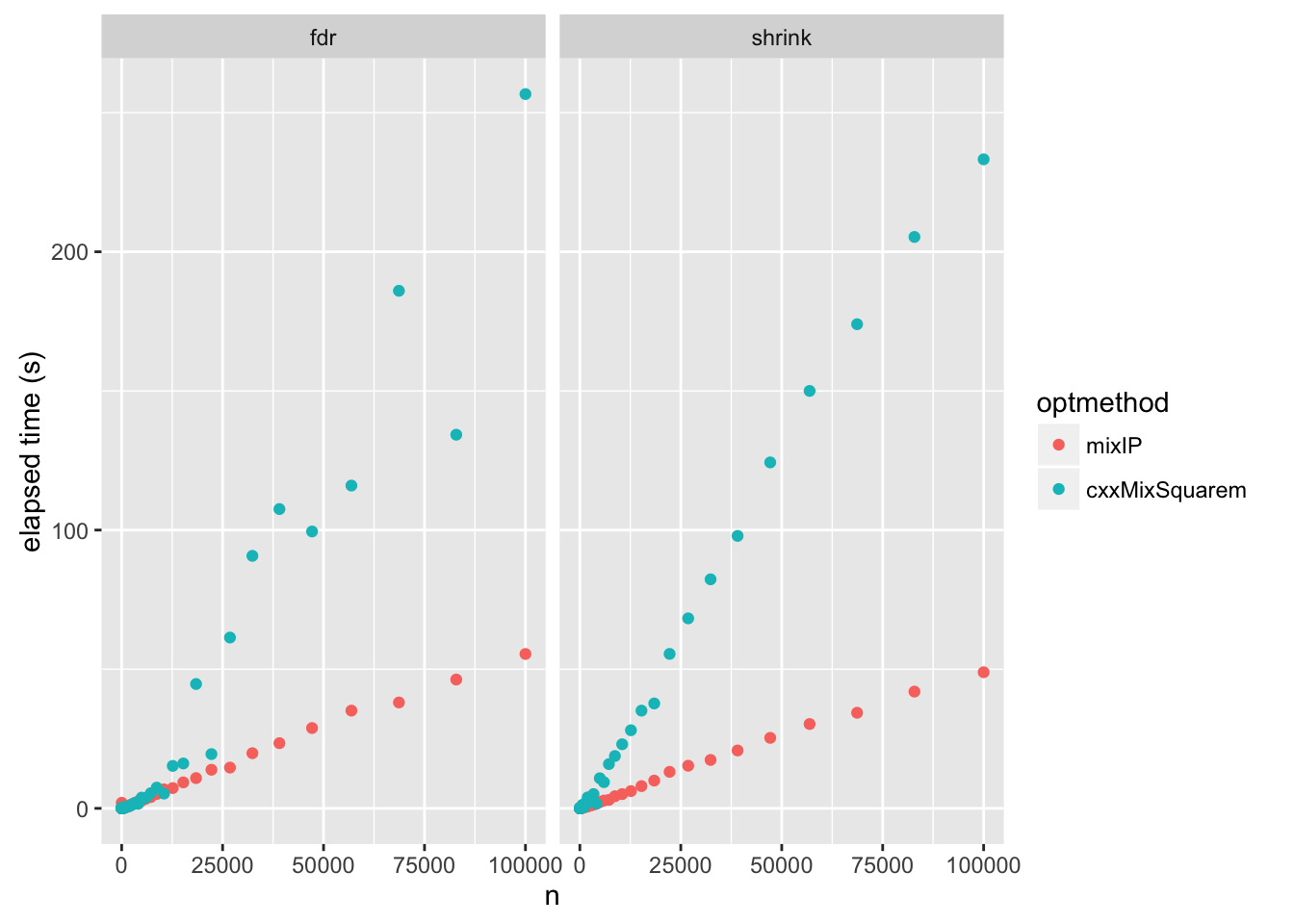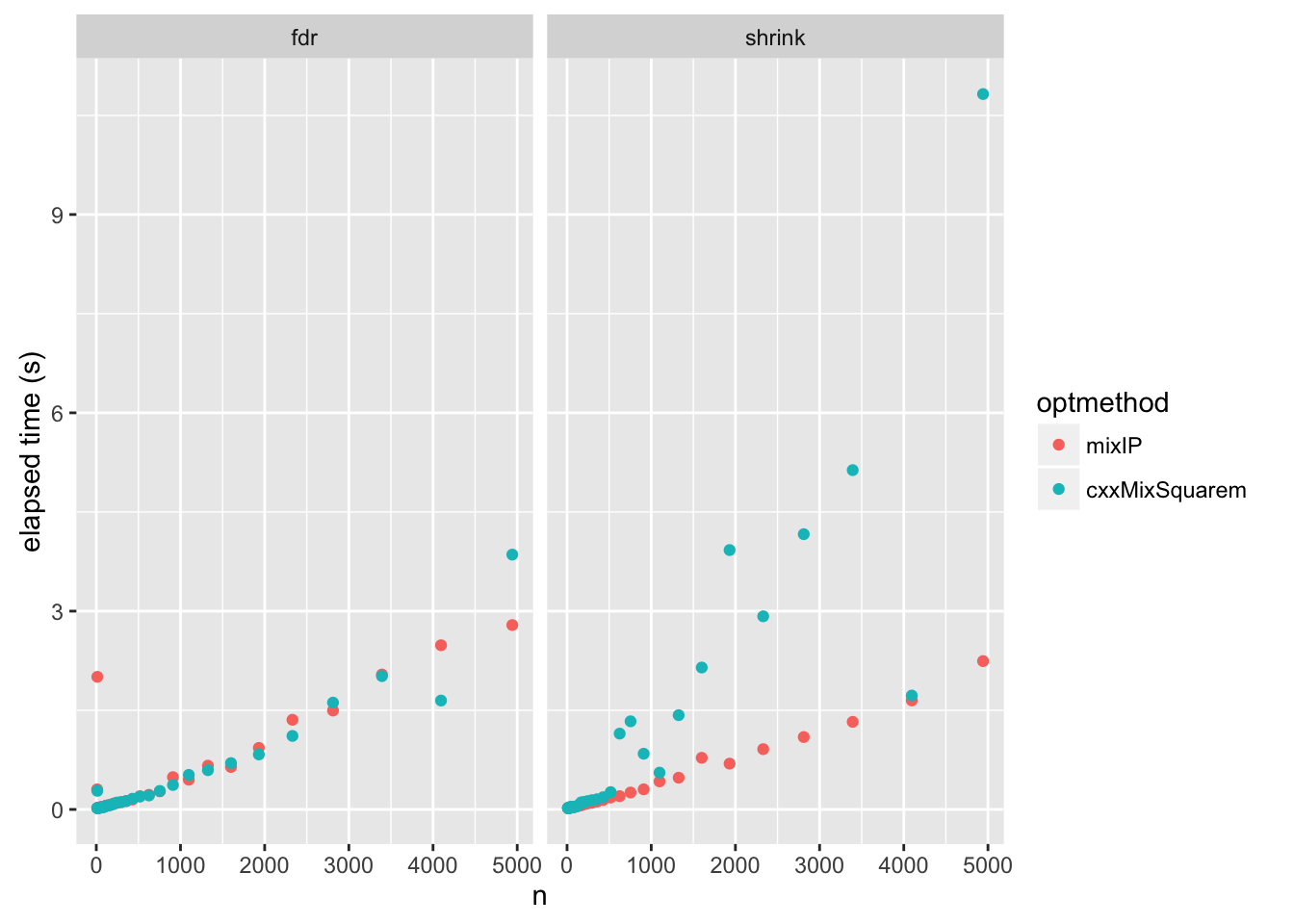Last updated: 2017-01-17

Code version: efcd39d916a87f7322f9c515c668905c36a86f9a

First, we load the necessary libraries.

library(REBayes)
## Loading required package: Matrix
library(ashr)
library(ggplot2)

## Set up simulation

The following simulation assumes $$\beta \sim N(0,\sigma_{\sf betasd}^2)$$ and with $$\hat{\beta}$$ having standard deviation 1.

timed_sims = function(ash.args,nsim = 20,nmin = 100,nmax = 1e3,betasd = 1) {
n = 10^seq(log10(nmin),log10(nmax),length = nsim)
elapsed.time = rep(0,nsim)
for (i in 1:nsim) {
set.seed(i)
with(ash.args,cat(sprintf("%6s %13s %3d\n",method,optmethod,i)))
betahat = rnorm(n[i],sd = sqrt(1 + betasd^2))
elapsed.time[i] =
system.time(do.call(ash,args = modifyList(ash.args,
list(betahat = betahat, sebetahat = 1))))
}
return(data.frame(elapsed.time = elapsed.time,seed = 1:nsim,n = n))
}

Now run a simulation study, for $$n$$ (number of tests; $$p$$ in paper) in range 10 to 100,000. Note that the warnings are being generated by the EM algorithm in big problems due to lack of convergence.

df = data.frame()
cat("method --optmethod-- sim\n")
for(method in c("fdr","shrink")) {
for(optmethod in c("mixIP","cxxMixSquarem")) {
df = rbind(df,data.frame(method = method,optmethod = optmethod,
timed_sims(list(method = method,optmethod = optmethod),
nsim = 50,nmin = 10,nmax = 1e5)))
}
}
Warning in estimate_mixprop(data, g, prior, optmethod = optmethod, control
= control): Optimization failed to converge. Results may be unreliable. Try
increasing maxiter and rerunning.

Warning in estimate_mixprop(data, g, prior, optmethod = optmethod, control
= control): Optimization failed to converge. Results may be unreliable. Try
increasing maxiter and rerunning.

Warning in estimate_mixprop(data, g, prior, optmethod = optmethod, control
= control): Optimization failed to converge. Results may be unreliable. Try
increasing maxiter and rerunning.

Warning in estimate_mixprop(data, g, prior, optmethod = optmethod, control
= control): Optimization failed to converge. Results may be unreliable. Try
increasing maxiter and rerunning.

Warning in estimate_mixprop(data, g, prior, optmethod = optmethod, control
= control): Optimization failed to converge. Results may be unreliable. Try
increasing maxiter and rerunning.

Warning in estimate_mixprop(data, g, prior, optmethod = optmethod, control
= control): Optimization failed to converge. Results may be unreliable. Try
increasing maxiter and rerunning.

Warning in estimate_mixprop(data, g, prior, optmethod = optmethod, control
= control): Optimization failed to converge. Results may be unreliable. Try
increasing maxiter and rerunning.

Warning in estimate_mixprop(data, g, prior, optmethod = optmethod, control
= control): Optimization failed to converge. Results may be unreliable. Try
increasing maxiter and rerunning.

Warning in estimate_mixprop(data, g, prior, optmethod = optmethod, control
= control): Optimization failed to converge. Results may be unreliable. Try
increasing maxiter and rerunning.

Warning in estimate_mixprop(data, g, prior, optmethod = optmethod, control
= control): Optimization failed to converge. Results may be unreliable. Try
increasing maxiter and rerunning.

Warning in estimate_mixprop(data, g, prior, optmethod = optmethod, control
= control): Optimization failed to converge. Results may be unreliable. Try
increasing maxiter and rerunning.

Warning in estimate_mixprop(data, g, prior, optmethod = optmethod, control
= control): Optimization failed to converge. Results may be unreliable. Try
increasing maxiter and rerunning.

Warning in estimate_mixprop(data, g, prior, optmethod = optmethod, control
= control): Optimization failed to converge. Results may be unreliable. Try
increasing maxiter and rerunning.

Warning in estimate_mixprop(data, g, prior, optmethod = optmethod, control
= control): Optimization failed to converge. Results may be unreliable. Try
increasing maxiter and rerunning.

Warning in estimate_mixprop(data, g, prior, optmethod = optmethod, control
= control): Optimization failed to converge. Results may be unreliable. Try
increasing maxiter and rerunning.

Warning in estimate_mixprop(data, g, prior, optmethod = optmethod, control
= control): Optimization failed to converge. Results may be unreliable. Try
increasing maxiter and rerunning.

Warning in estimate_mixprop(data, g, prior, optmethod = optmethod, control
= control): Optimization failed to converge. Results may be unreliable. Try
increasing maxiter and rerunning.

Warning in estimate_mixprop(data, g, prior, optmethod = optmethod, control
= control): Optimization failed to converge. Results may be unreliable. Try
increasing maxiter and rerunning.

Warning in estimate_mixprop(data, g, prior, optmethod = optmethod, control
= control): Optimization failed to converge. Results may be unreliable. Try
increasing maxiter and rerunning.
cat("\n")

Summarize the model fitting results in a table.

cat("Average computation time (in seconds):\n")
Average computation time (in seconds):
print(as.table(by(df,df[c("method","optmethod")],
function (x) mean(x\$elapsed.time))))
        optmethod
method    mixIP cxxMixSquarem
fdr     6.800        23.699
shrink  5.972        27.931

## Results

Now plot time as a function of $$n$$:

qplot(x = n,y = elapsed.time,data = df,col = optmethod,facets = .~method,
ylab = "elapsed time (s)")Zoom-in on “small” problems with $$n < 5000$$.

qplot(x = n,y = elapsed.time, data = subset(df,n < 5000),
col = optmethod,facets = .~method,ylab = "elapsed time (s)")## Summary

The IP method clearly scales to large problem much better than EM. It is faster, and also more reliable (sometimes reaches higher log-likelihood than EM, never smaller); see checkIP.html.

However, for small problems (n < 5000) the EM is adequate for many practical purposes, solving within a few seconds. This is particularly true for the penalty term (method = fdr), which helps the EM converge, presumably because it is helping identifiability, removing the large flat parts of the likelihood objective function.

Indeed, for small problems with the penalty term (n < 2000) EM with squarem acceleration is a little faster in these comparisons than IP.

## Session information

sessionInfo()
R version 3.3.2 (2016-10-31)
Platform: x86_64-apple-darwin13.4.0 (64-bit)
Running under: OS X El Capitan 10.11.6

locale:
 en_US.UTF-8/en_US.UTF-8/en_US.UTF-8/C/en_US.UTF-8/en_US.UTF-8

attached base packages:
 stats     graphics  grDevices utils     datasets  methods   base

other attached packages:
 knitr_1.14     ggplot2_2.1.0  ashr_2.0.4     REBayes_0.63
 Matrix_1.2-7.1

loaded via a namespace (and not attached):
 Rcpp_0.12.8       magrittr_1.5      MASS_7.3-45
 munsell_0.4.3     doParallel_1.0.10 pscl_1.4.9
 colorspace_1.2-7  SQUAREM_2016.8-2  lattice_0.20-34
 foreach_1.4.3     plyr_1.8.4        stringr_1.1.0
 tools_3.3.2       parallel_3.3.2    grid_3.3.2
 gtable_0.2.0      htmltools_0.3.5   iterators_1.0.8
 assertthat_0.1    yaml_2.1.13       rprojroot_1.1
 digest_0.6.10     reshape2_1.4.1    formatR_1.4
 codetools_0.2-15  evaluate_0.10     rmarkdown_1.2
 labeling_0.3      stringi_1.1.2     Rmosek_7.1.3
 scales_0.4.0      backports_1.0.3   truncnorm_1.0-7